Tip: Other languages are Google-Translated. You can visit the English version of this link.

## Lookup values from another worksheet or workbook

If you know how to use VLOOKUP function to search for values in a worksheet, vlookup values from another worksheet or workbook won’t be a problem for you.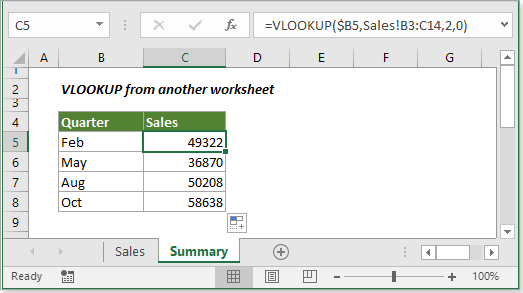#### VLOOKUP values from another worksheet

This section is going to show you how to vlookup values from another worksheet in Excel.

Generic formula

=VLOOKUP(lookup_value,sheet_range,col_index,[range_lookup])

Arguments

• Lookup_value (required): The value you are searching for. It must be in the first column of the sheet_range.
• Sheet_range (required): A range of cells in a certain worksheet which contains two or more columns where the lookup value column and the result value column locating.
• Col_index (required): The specific column number (it is an integer) of the table_array, which you will return the matched value from.
• Range_lookup (optional): This is a logical value that determines whether this VLOOKUP function will return an exact match or an approximate match.

Click to know more about the VLOOKUP function.

In this case, I need to lookup values in the range B3:C14 of the worksheet named “Sales”, and return the corresponding results in the Summary worksheet.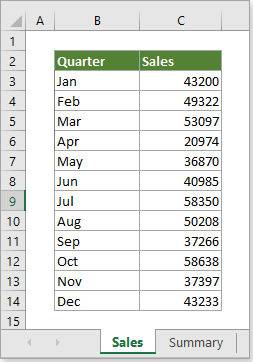1. Select a blank cell in the Summary worksheet, copy the below formula into it and press the Enter key.

=VLOOKUP(\$B5,Sales!B3:C14,2,0)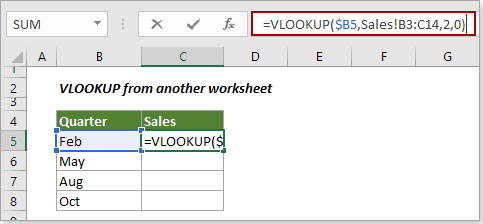Notes:

• B5 is the cell reference containing the value you are searching for;
• Sales is the sheet name you will lookup value from;
• B3:C14 is the range contains the lookup values column and the result values column;
• 2 means that the result value locating on the second column of range B3:C14;
• 0 here means that the VLOOKUP function will return an exact match. If an exact match value can’t be found, it will return a #N/A error value.

2. Then drag the Fill Handle down to get all results.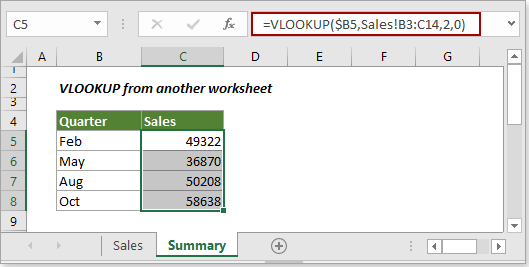#### VLOOKUP values from another workbook

Assuming there is a wokbook name "Sales report", for directly lookup values in a specific sheet of this workbook even it is closed, please do as follows.

Generic formula

=VLOOKUP(lookup_value,[workbook]sheet!range,col_index,[range_lookup])

Arguments

• Lookup_value (required): The value you are searching for. It must be in the first column of the sheet_range.
• [workbook]sheet!range (required): The range of cells of a sheet in specific workbook, which contains two or more columns where the lookup value column and the result value column locating.
• Col_index (required): The specific column number (it is an integer) of the table_array, which you will return the matched value from.
• Range_lookup (optional): This is a logical value that determines whether this VLOOKUP function will return an exact match or an approximate match.

1. Select a blank cell, copy the below formula into it and press the Enter key.

=VLOOKUP(\$B5,'[Sales report.xlsx]Sales'!B3:C14,2,0)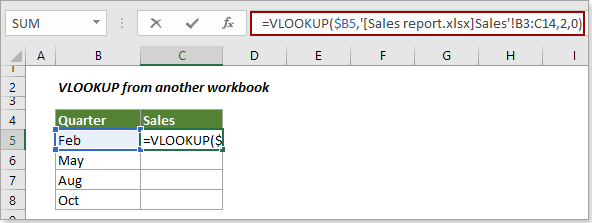Note: If an exact match value can’t be found, The VLOOKUP function will return a #N/A error value.

2. Then drag the Fill Handle down to apply the formula to other cells to get all results. See screenshot: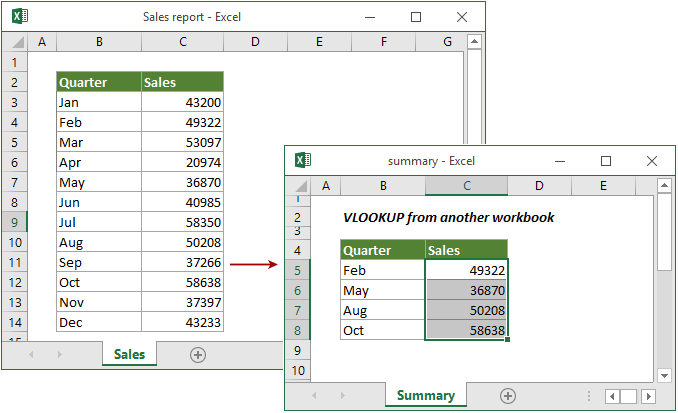#### Related function

The VLOOKUP function
The Excel VLOOKUP function searches for a value by matching on the first column of a table and returns the corresponding value from a certain column in the same row.

#### Related formulas

Vlookup formula – the shipping cost calculator
This article provides detailed steps to calculate shipping cost based on specified weight of an item in Excel.
Click to know more...

Lookup values across multiple worksheets with dynamic sheet name
In many cases, you may need to collect data across multiple worksheets for summary. This article provides methods for you to easily lookup specific values across worksheets with dynamic sheet name.
Click to know more...

Vlookup and return matched values in multiple columns
Normally, applying the Vlookup function can only return the matched value from one column. Sometimes, you may need to extract matched values from multiple columns based on the criteria. Here is the solution for you.
Click to know more...

Vlookup to return multiple values in one cell
Normally, when applying the VLOOKUP function, if there are multiple values that match the criteria, you can only get the result of the first one. If you want to return all matched results and display them all in a single cell, how can you achieve?
Click to know more...

Vlookup and return entire row of a matched value
Normally, using the vlookup function can only return a result from a certain column in the same row. This article is going to show you how to return the whole row of data based on specific criteria.
Click to know more...

### Excel Productivity Tools

Would you like to complete your daily work quickly and perfectly? Kutools for Excel brings 300 powerful advanced features (Combine workbooks, sum by color, split cell contents, convert date, and so on...) and save 70% time.

• Designed for 1500 work scenarios, helps you solve 80% Excel problems.
• Reduce thousands of keyboard and mouse clicks every day, relieve your tired eyes and hands.
• Become an Excel expert in 3 minutes. No longer need to remember any painful formulas and VBA codes.
• 60-day unlimited free trial. 60-day money back guarantee. Free upgrade and support for 2 years.

#### Brings Efficient Tabs to Office (include Excel), Just Like Chrome, Firefox, And New IE

• Office Tab Increases your productivity by 50% when viewing and editing multiple documents.
• Reduce hundreds of mouse clicks for you every day, say goodbye to mouse hand.
• One second to switch between dozens of open documents!Say something here...
symbols left.